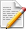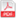﻿

#### 当期目录

1996年 第17卷 第12期    刊出日期：1996-12-18
论文
ON THE GENERAL SOLUTION OF CYLINDRICAL SHELL EQUATIONS
薛毅;薛大为
1996, 17(12):  1107-1112.摘要 ( 494 )PDF (347KB) ( 370 )
参考文献 | 相关文章 | 多维度评价
It is proved mathematically in this paper that the strain-stress function F(ξ φ) on the cylindrical shell theory suggested by Vlasov  will give out the general solution of the simultaneous partial differential equations of cylindrical shell problem. That is to say. there is no any solution Of the simultaneous partial differential equations can be omitted due to Vlasov's suggestion. The conclusion suggested in this paper is helpful to the well-known Vlasov's method.
FIXED POINT THEOREMS FOR FUZZY MAPPINGS IN PROBABILISTIC METRIC SPACES
张石生;张颖;袁家玮
1996, 17(12):  1113-1120.摘要 ( 524 )PDF (574KB) ( 676 )
参考文献 | 相关文章 | 多维度评价
In this paper some fuzzy fixed point theorems and fixed degree theorems areobtained under the framework of probabilistic metric space, which contain and improvesome recent results.
NEAR CRACK LINE ELASTIC-PLASTIC ANALYSIS FOR A CRACK LOADED BY ANTIPLANE POINT FORCES
吴承平;王成
1996, 17(12):  1121-1126.摘要 ( 484 )PDF (398KB) ( 424 )
参考文献 | 相关文章 | 多维度评价
In this paper, the improved near crack line analysis method proposed in Refs. and  is used to investigate a mode Ⅲ crack loaded by antiplane point forces in aninfinite plate in an elastic-perfectly plastic solid. The solutions of this paper aresufficiently precise near the crack line region because. the assumptions of the smallscale yielding theory have not been used and no other assumptions have been taken.
ANALYSIS OF OSCILLATORY FLOW IN CONSIDERATION OF A PLASMA LAYER IN ARTERIAL STENOSES
王长斌;柳兆荣
1996, 17(12):  1127-1135.摘要 ( 500 )PDF (544KB) ( 558 )
参考文献 | 相关文章 | 多维度评价
This paper presents the influences of plasma layer on the oscillatory flow inarterial stenosis. The analysis demonstrates that the existence of the plasma layer mayobviously change the characteristics of flow such as velocity-profiles, longitudinalimpedance and pressure gradient, but hardly change the phase of longitudinalimpedance and pressure gradient. Besides. such influences vary with a and degree ofstenosis. These analyses have Special physiological significance in blood circulationsystem.
APPLICATION OF VECTOR TO SMALL DEFORMATION
张慎学
1996, 17(12):  1137-1140.摘要 ( 491 )PDF (287KB) ( 321 )
参考文献 | 相关文章 | 多维度评价
This paper first gives the definition of strain vector in elasticity body which is insmall deformation, and points out that the strain state of point in the body is describedby projection forms of the strain vector at this point. and then gives relation betweenstrain vector-stress vector for isotropic elasticity body, moreover derives conditionsthat strain vector coincides with stress vector and the material is incompressible.
THE THEORETICAL COST OF SEQUENTIAL AND PARALLEL ALGORITHMS FOR SOLVING LINEAR SYSTEMS OF EQUATIONS
Salman H. Abbas
1996, 17(12):  1141-1146.摘要 ( 388 )PDF (405KB) ( 350 )
参考文献 | 相关文章 | 多维度评价
In this paper two parallel algorithms for solving dense linear equations arediscussed. The algorithms are based on LU-decomposition followed by forward andbackward substitutions. The algorithms are numerically stable and have been testedon the Sequent Balance Machine with efficient utilization of all processors.
ON THE SECOND ORDER WAVE DIFFRACTION IN TWO LAYER FLUIDS
吴建华;方颖
1996, 17(12):  1147-1152.摘要 ( 511 )PDF (510KB) ( 356 )
参考文献 | 相关文章 | 多维度评价
In this paper, with assumption that the stratified ocean consists of two layer fluidswith different densities, the problem of the second order wave diffraction by threedimensional bodies in the stratifed ocean is investigated With the use of the regularperturbation method. the boundary value problem of the second order multi-chromaticwave scattering potential is firstly formulated, and is based on a weakly radiationcondition, a formal solution Of this boundary value problem is then found. By usingGreen theorem and introducing an assisting potential, the integral expressions, whichdo not explicitly connect with the second order scattering potential, of the second orderwave loads are also derived. Our analysis indicates that the effects of the stratificationupon the second order difference frequency wave loads on the structures may besignificant.
BENDING SOLUTION OF A RECTANGULAR PLATE WITH ONE EDGE BUILT-IN AND ONE CORNER POINT SUPPORTED SUBJECTED TO UNIFORM LOAD
许琪楼;姬同庚
1996, 17(12):  1153-1163.摘要 ( 441 )PDF (605KB) ( 481 )
参考文献 | 相关文章 | 多维度评价
In this paper, using of the superposition principle. the bending solution ofrectangular plate with one edge built-in and one corner point supported subjected touniform load is derived. The results indicate the method has the advantages of rabidconvergence and high precision.
ANOTHER CLASS D'ALEMBERT PRINCIPLE AND A NEW MAGGI EQUATION FOR ARBITRARY ORDER NONHOLONOMIC MECHANICAL SYSTEMS IN DERIVATIVE SPACE
张曙红;梁天麟
1996, 17(12):  1165-1169.摘要 ( 386 )PDF (392KB) ( 347 )
参考文献 | 相关文章 | 多维度评价
As a concrete application of the concepts of "derivative space" and"correspondent kinetic energy" in derivative space, and of foe thought of "treatingnonholonomic systems by changing them into formal holonomic system" which theauthors have previously proposed in references [1, 2, 3]. this paper derived another newuniversal D'Alembert principle and a new Maggi equation for arbitrary ordernonholonomic mechanical systems. An example using the Maggi equation is given.
STABILITY OF NON-CONSERVATIVE LINEAR GYROSCOPIC SYSTEMS
李俊峰;王照林
1996, 17(12):  1171-1175.摘要 ( 454 )PDF (361KB) ( 611 )
参考文献 | 相关文章 | 多维度评价
The paper investigates the stability of linear non-conservative mechanical systemssubjected to potential. gyroscopic, circulatory forces and Rayleigh damping. Threestability theorems are proved by means of the Rayleigh quotients. The stabilitycriterions given by, the theorems are convenient and useful because they areindependent of the Rayleigh quotients.
STUDIES OF MELNIKOV METHOD AND TRANSVERSAL HOMOCLINIC ORBITS IN THE CIRCULAR PLANAR RESTRICTED THREE-BODY PROBLEM
朱如曾;向程
1996, 17(12):  1177-1187.摘要 ( 422 )PDF (727KB) ( 483 )
参考文献 | 相关文章 | 多维度评价
Non-Hamiltonian systems containing degenerate fixed points obtained from twodegrees of freedom near-integrable Hamiltonian systems through non-canonicaltransformations are dealt with in this paper. Two criteria.for determining theexistence of transversal homoclinic and heteroclinic orbits are presented. By exploitingthese criteria the existence of the transversal homoclinic orbits and so, of thetransversal homoclinic tangle.phenomenon in the near-integrable circular planarrestricted three-body problem with sufficiently small mass ratio of the two primaries isproven. Under some assumptions, the existence of the transversal heleroclinic orbits isproven. The global qualitative phase diagram is also illustrated.
AN EXACT METHOD OF BENDING OF ELASTIC THIN PLATES WITH ARBITRARY SHAPE
周叮
1996, 17(12):  1189-1192.摘要 ( 399 )PDF (284KB) ( 330 )
参考文献 | 相关文章 | 多维度评价
This paper presents a new method exactly to solve the bending of elastic thinplates with arbitrary shape. First the analytic solution of differential equation ofelastic thin plate is derived in polar coordinate, then the analytic solution is substituted into the boundary conditions of elastic thin plate with arbitrary shape. The boundaryequations are expanded along the boundary by the use of Fourier series, all unknown coefficients can be decided. The results are exact.
SINGULAR PERTURBATIONS FOR A CLASS OF BOUNDARY VALUE PROBLEMS OF HIGHER ORDER NONLINEAR DIFFERENTIAL EQUATIONS
史玉明;刘光旭
1996, 17(12):  1193-1201.摘要 ( 416 )PDF (784KB) ( 409 )
参考文献 | 相关文章 | 多维度评价
In this paper, it has been studied that the singular perturbations for the higherorder nonlinear boundary value problem of the formε2y(n)=f(t, ε, y. "', y(n-2))pj(ε)y(1)(0, ε)-qj(ε)y(j+1)(0. ε)=Aj(ε) (0≤j≤n-3)a1(ε)u(n-2)(0.ε)-a2(ε)y(n-1)(0, ε)=B(ε)b1(ε)y(n-2)(1, ε)+b2(ε)y(n-1),(1. ε)=C(ε)by the method of higher order differential inequalities and boundary layer corrections.Under some mild conditions, the existence of the perturbed solution is proved and itsuniformly efficient asymptotic expansions up to its n-th order derivative function aregiven out. Hence, the existing results are extended and improved.
[an error occurred while processing this directive]